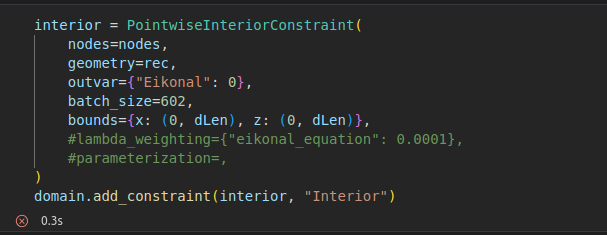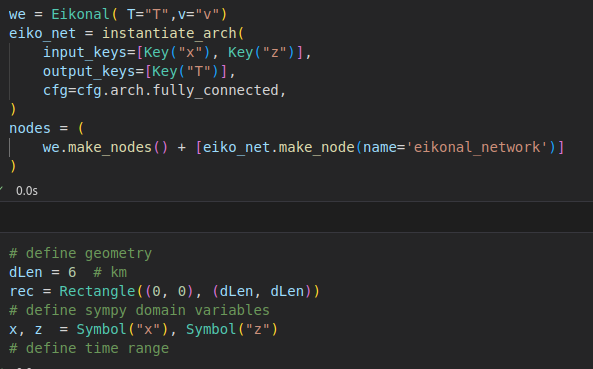# SymPy size error and Setting 2D point constraints

I’m trying to implement this PDE class and I’m having some difficulties, am I doing something wrong?

At the PointwiseInteriorConstraint I get the error: ValueError: C order implies last dim (1) == len(args) (2)error:

I need the initial condition T(4,1) = 0 and I am not successfulhow the network was setI would be grateful if someone could help me.

I’m not sure what is causing that error you’re getting. I was not able to reproduce it on my end. However there are a few issues. The first is that the `Rectangle` geometry object returns `x,y`, so your ‘z’ variable in your PDE and model won’t be provided. This would cause a graph unrolling error. The fix is simply changing `z` to `y`.

The second issue is that in your `PointwiseInteriorConstraint` you don’t provide a `v` variable which will also cause a graph unrolling error. Your PDE can’t be calculated without it. To fix this I added `v` as a parameterized variable.

I created a simple script to test on my end and seems it works fine on my end:

``````class Eikonal(PDE):
name = "Eikonal"
def __init__(self, T="T", v="v"):
self.T = T
x, y = Symbol('x'), Symbol('y')
input_variables = {"x": x, "y": y}
T = Function(T)(*input_variables)
v = Function(v)(*input_variables)

self.equations = {}
self.equations['Eikonal'] = ((T.diff(x))**2 + (T.diff(y))**2 + (1/v)**2)

@modulus.sym.main(config_path="conf", config_name="config")
def run(cfg: ModulusConfig) -> None:
# make list of nodes to unroll graph on
pde = Eikonal( T="T", v="v")
model = instantiate_arch(
input_keys=[Key("x"), Key("y")],
output_keys=[Key("T")],
cfg=cfg.arch.fully_connected,
)
nodes = pde.make_nodes() + [model.make_node(name="my_network")]

dLen = 6
rec = Rectangle((0,0), (dLen, dLen))
# make geometry
x, y = Symbol("x"), Symbol("y")
v = Symbol("v")

interior = PointwiseInteriorConstraint(
nodes=nodes,
geometry=rec,
outvar={"Eikonal": 0},
batch_size=128,
parameterization={v: 0}
)
# ..... more code here

if __name__ == "__main__":
run()
``````
1 Like

@ngeneva

Dear @ngenevam I’m really grateful for help me with the code, now it is working. However, I would like to ask for another question. Looking in the documentation, I can’t find a good way to make a point restriction on data in two dimensions of two dimensions. I mean, in this case I need T(x,y) to be like this T(4,1) = (0,0). I looked at the criteria but it refers to boundary conditions my problem has no border. I tried this way but I was not successful,

``````font = PointwiseInteriorConstraint(
nodes = nodes,
geometry = rec,
outvar={"T":0.0},
batch_size = 1,
parameterization= {x:1 , y:4 },

)

``````

or more easy T = 0 for v = 0 using PointwiseConstraint.from_numpy
Could you help me with this?

Yes, unfortunately we don’t have a 2D point primitive as far as I know. You could try a few things.

1. Define the input/ output training points for this constraint manually via numpy dictionaries. (See this thread and embedded links). This is probably what I would recommend the most.

2. Use the criteria to create a small bounding box around the point of interest.

3. A hacky approach would be to use a 1D point geometry for the x dimension and then parameterize the y variable like what you have here.

4. Another option I would try is to create a 2D circle geometry with a really small radius so its approximately a point.

1 Like

@ngeneva

Thanks. I was able to run successfully after the previous suggestion using PointwiseConstraint.from_numpy. I’ll try the other two suggestions as I need to vary this initial condition. A suggestion for Nvidia is to generate the direct possibility of entering these point restriction conditions

This topic was automatically closed 14 days after the last reply. New replies are no longer allowed.